# NCERT Exemplar Class 11 Chemistry Solutions for Chapter 10 - The S Block Elements

## NCERT Exemplar Solutions Class 11 Chemistry Chapter 10 – Free PDF Download

NCERT Exemplar Solutions for Chemistry Class 11 Chapter 10 The s-Block Elements is a crucial study material you can rely on to prepare for the CBSE Class 11 Chemistry and other entrance examinations. The NCERT Exemplar Class 11 Chemistry Chapter 10 consists of answers to questions given in the NCERT Exemplar book, along with extra questions on the chapter s-Block Elements prepared by the subject expert at BYJU’S. This solution also consists of the important questions from previous years’ question papers and sample papers.

In this chapter, students will learn about the elements which are present in S-block. The elements present in the s-block are hydrogen (H), helium (He), lithium (Li), beryllium (Be), sodium (Na), magnesium (Mg), potassium (K), calcium (Ca), rubidium (Rb), strontium (Sr), caesium (Cs), barium (Ba), francium (Fr) and radium (Ra).

### Download the PDF of the NCERT Exemplar for Class 11 Chemistry Chapter 10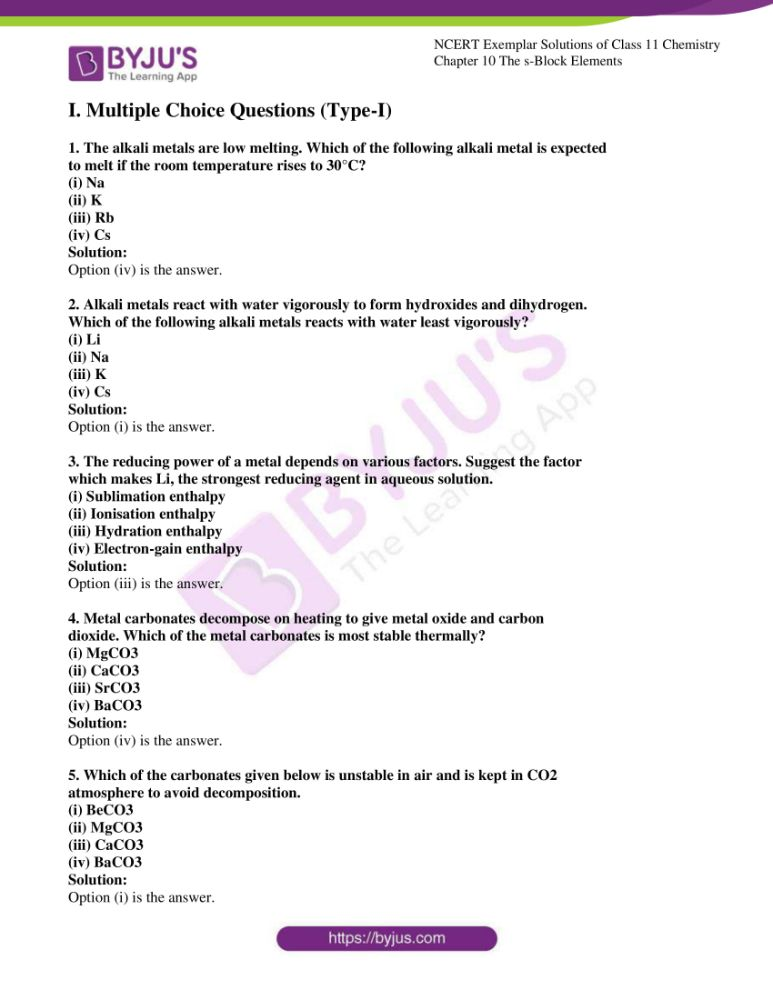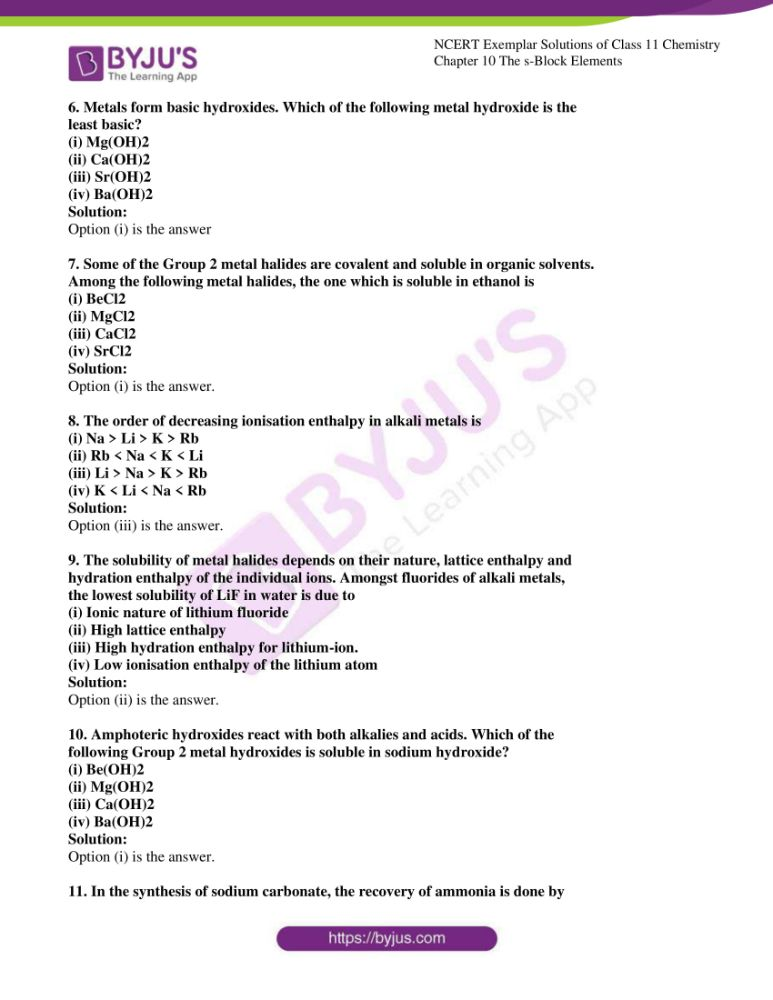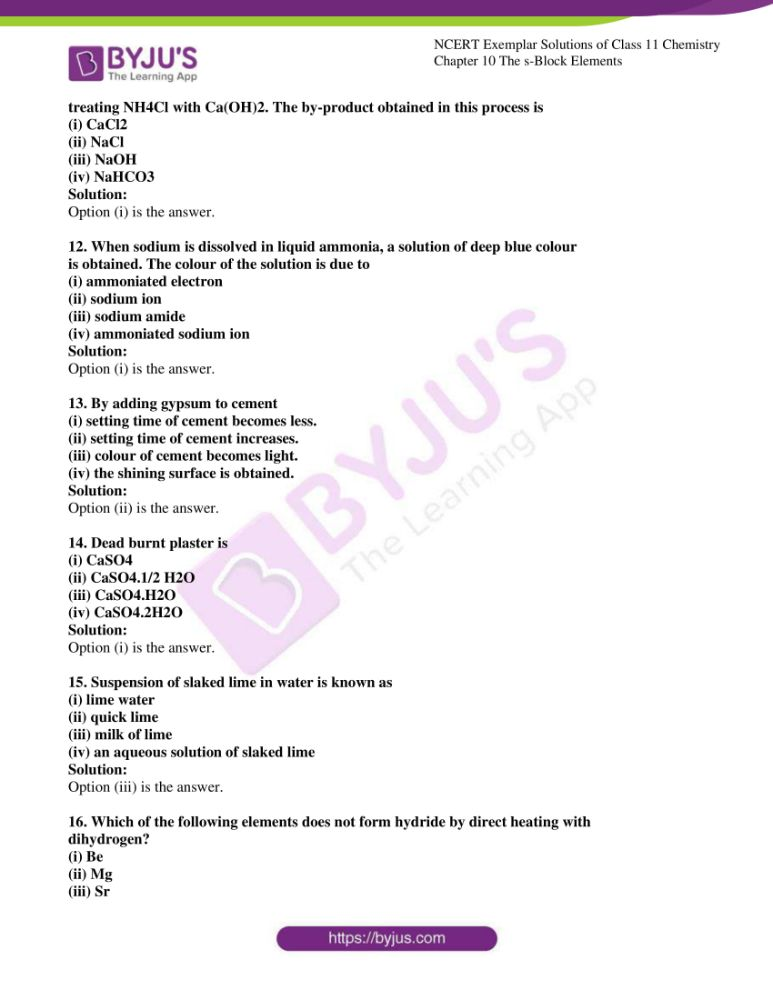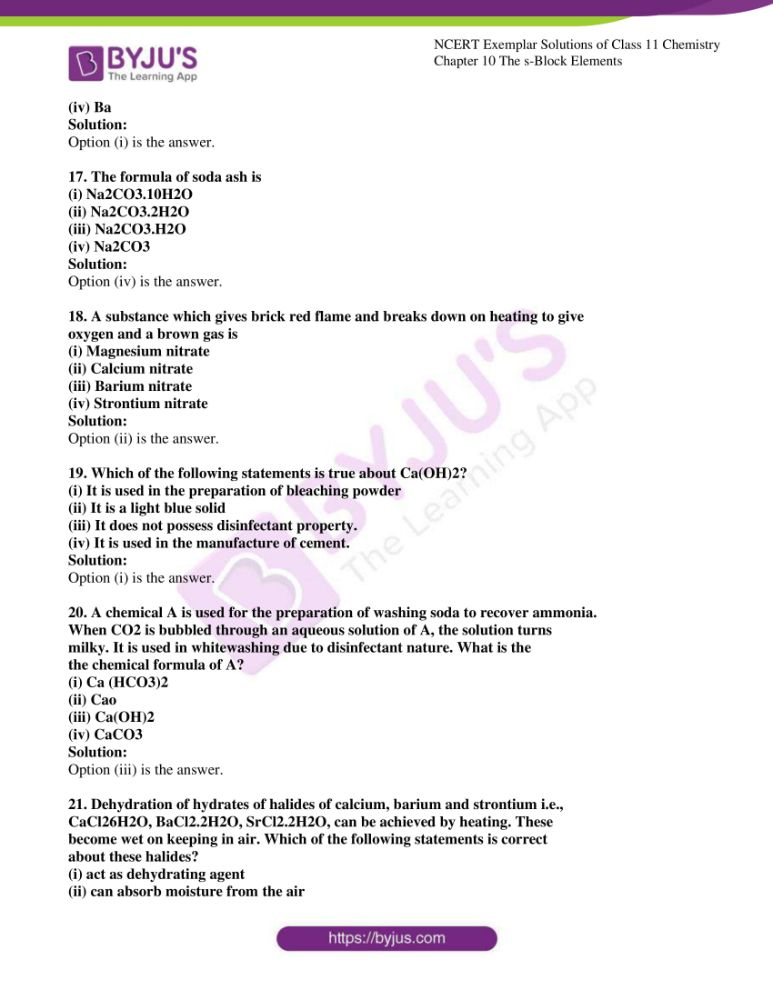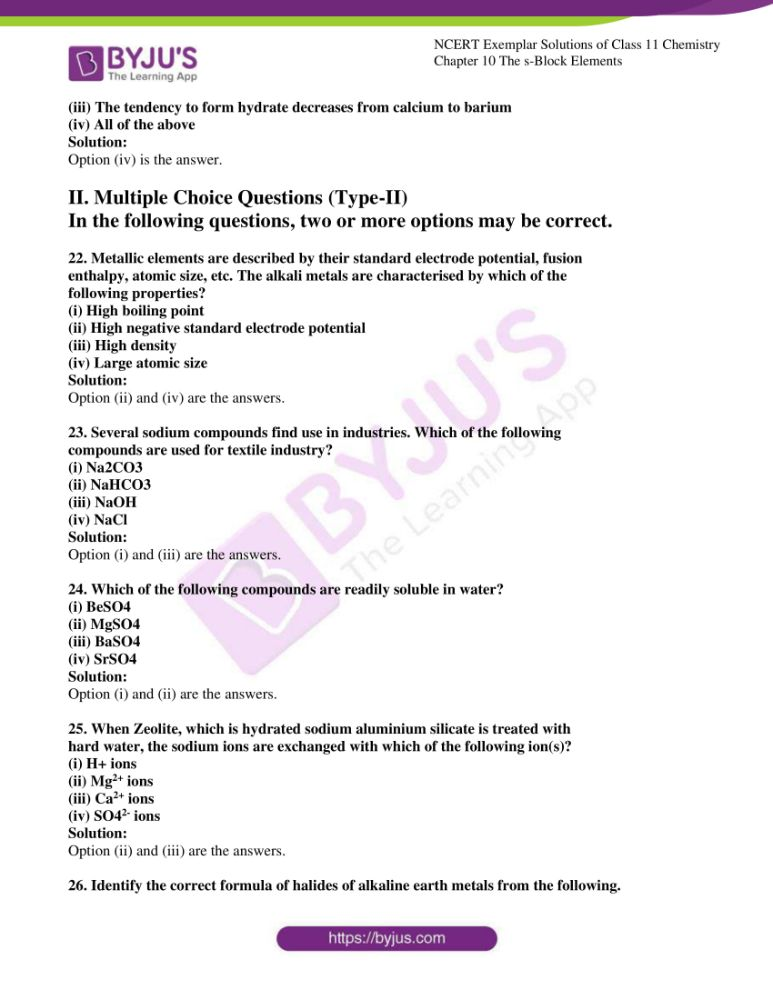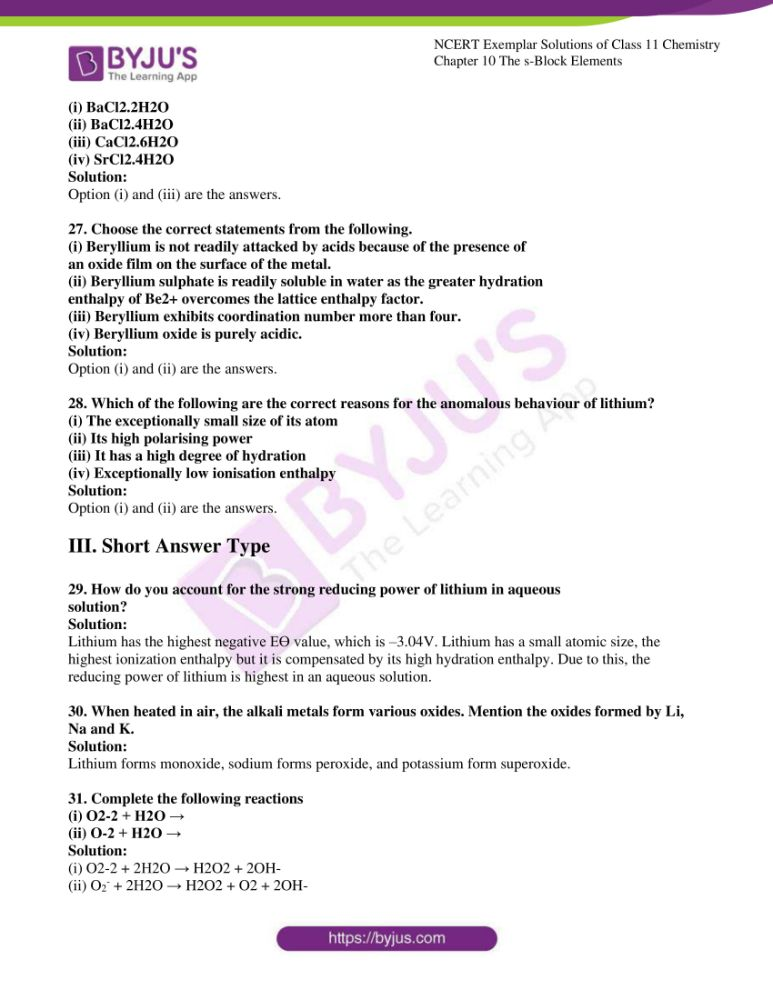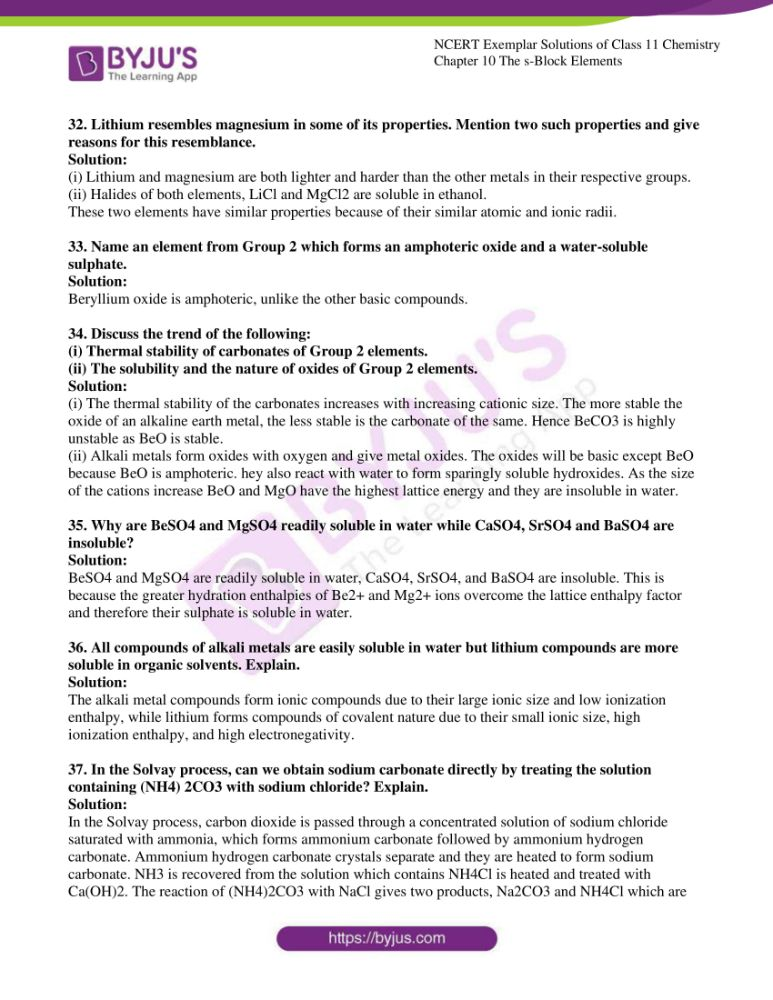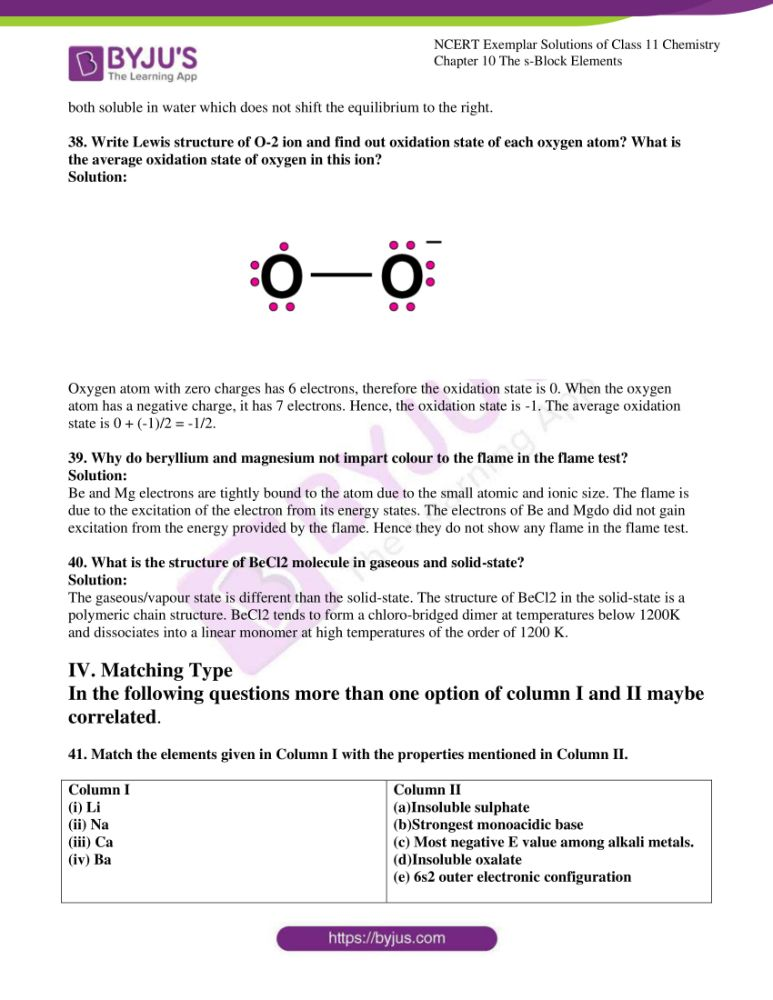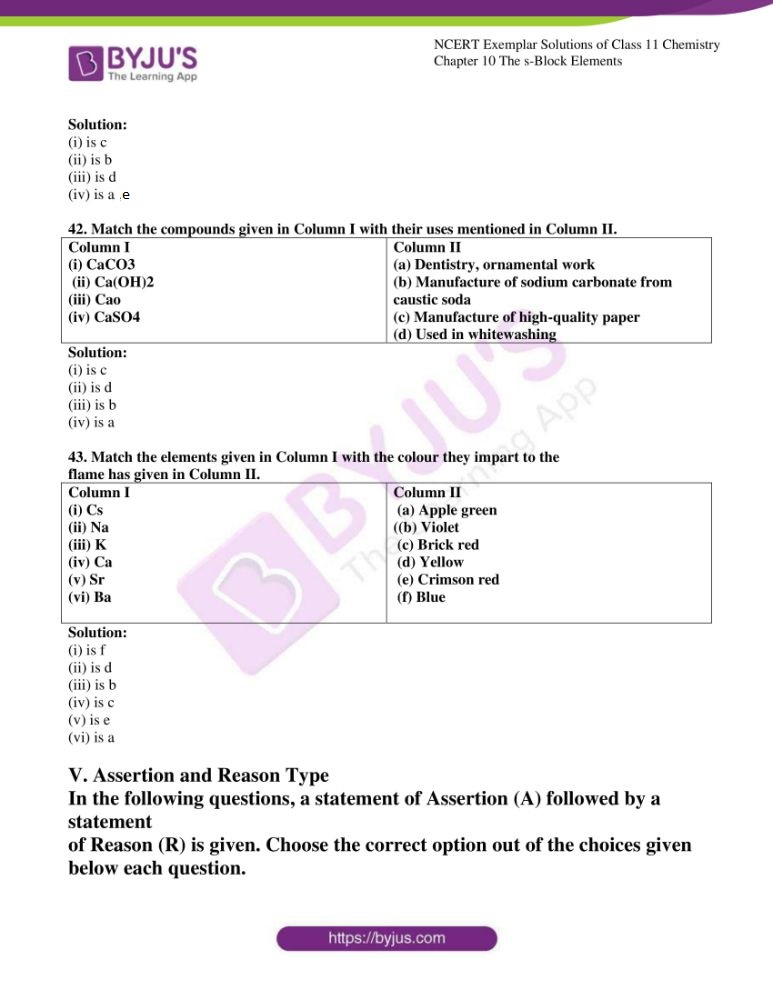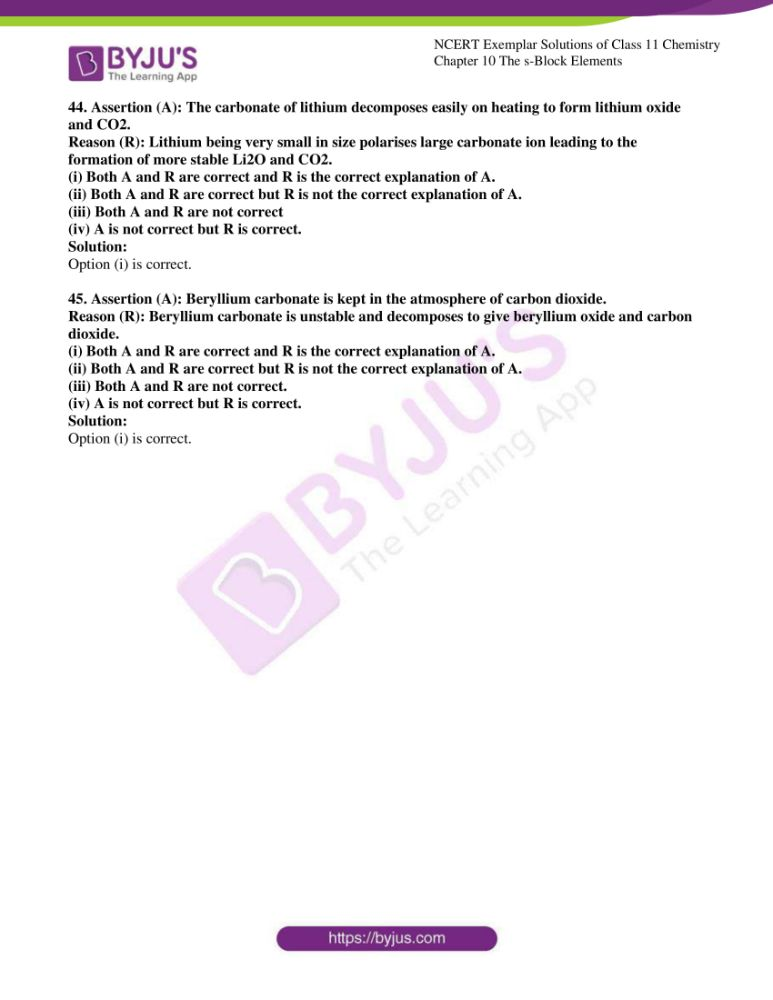### Access Answers to NCERT Exemplar Solutions for Class 11 Chemistry Chapter 10

I. Multiple Choice Questions (Type-I)

1. The alkali metals are low melting. Which of the following alkali metal is expected

to melt if the room temperature rises to 30°C?

(i) Na

(ii) K

(iii) Rb

(iv) Cs

Solution:

2. Alkali metals react with water vigorously to form hydroxides and dihydrogen.

Which of the following alkali metals reacts with water least vigorously?

(i) Li

(ii) Na

(iii) K

(iv) Cs

Solution:

3. The reducing power of a metal depends on various factors. Suggest the factor

which makes Li, the strongest reducing agent in aqueous solution.

(i) Sublimation enthalpy

(ii) Ionisation enthalpy

(iii) Hydration enthalpy

(iv) Electron-gain enthalpy

Solution:

4. Metal carbonates decompose on heating to give metal oxide and carbon

dioxide. Which of the metal carbonates is most stable thermally?

(i) MgCO3

(ii) CaCO3

(iii) SrCO3

(iv) BaCO3

Solution:

5. Which of the carbonates given below is unstable in air and is kept in CO2

atmosphere to avoid decomposition.

(i) BeCO3

(ii) MgCO3

(iii) CaCO3

(iv) BaCO3

Solution:

6. Metals form basic hydroxides. Which of the following metal hydroxide is the

least basic?

(i) Mg(OH)2

(ii) Ca(OH)2

(iii) Sr(OH)2

(iv) Ba(OH)2

Solution:

7. Some of the Group 2 metal halides are covalent and soluble in organic solvents.

Among the following metal halides, the one which is soluble in ethanol is

(i) BeCl2

(ii) MgCl2

(iii) CaCl2

(iv) SrCl2

Solution:

8. The order of decreasing ionisation enthalpy in alkali metals is

(i) Na > Li > K > Rb

(ii) Rb < Na < K < Li

(iii) Li > Na > K > Rb

(iv) K < Li < Na < Rb

Solution:

9. The solubility of metal halides depends on their nature, lattice enthalpy and

hydration enthalpy of the individual ions. Amongst fluorides of alkali metals,

the lowest solubility of LiF in water is due to

(i) Ionic nature of lithium fluoride

(ii) High lattice enthalpy

(iii) High hydration enthalpy for lithium-ion.

(iv) Low ionisation enthalpy of the lithium atom

Solution:

10. Amphoteric hydroxides react with both alkalies and acids. Which of the

following Group 2 metal hydroxides is soluble in sodium hydroxide?

(i) Be(OH)2

(ii) Mg(OH)2

(iii) Ca(OH)2

(iv) Ba(OH)2

Solution:

11. In the synthesis of sodium carbonate, the recovery of ammonia is done by

treating NH4Cl with Ca(OH)2. The by-product obtained in this process is

(i) CaCl2

(ii) NaCl

(iii) NaOH

(iv) NaHCO3

Solution:

12. When sodium is dissolved in liquid ammonia, a solution of deep blue colour

is obtained. The colour of the solution is due to

(i) ammoniated electron

(ii) sodium ion

(iii) sodium amide

(iv) ammoniated sodium ion

Solution:

13. By adding gypsum to cement

(i) setting time of cement becomes less.

(ii) setting time of cement increases.

(iii) colour of cement becomes light.

(iv) the shining surface is obtained.

Solution:

(i) CaSO4

(ii) CaSO4.1/2 H2O

(iii) CaSO4.H2O

(iv) CaSO4.2H2O

Solution:

15. Suspension of slaked lime in water is known as

(i) lime water

(ii) quick lime

(iii) milk of lime

(iv) an aqueous solution of slaked limeSolution:

16. Which of the following elements does not form hydride by direct heating with

dihydrogen?

(i) Be

(ii) Mg

(iii) Sr

(iv) Ba

Solution:

17. The formula of soda ash is

(i) Na2CO3.10H2O

(ii) Na2CO3.2H2O

(iii) Na2CO3.H2O

(iv) Na2CO3

Solution:

18. A substance which gives brick red flame and breaks down on heating to give

oxygen and a brown gas is

(i) Magnesium nitrate

(ii) Calcium nitrate

(iii) Barium nitrate

(iv) Strontium nitrate

Solution:

19. Which of the following statements is true about Ca(OH)2?

(i) It is used in the preparation of bleaching powder

(ii) It is a light blue solid

(iii) It does not possess disinfectant property.

(iv) It is used in the manufacture of cement.

Solution:

20. A chemical A is used for the preparation of washing soda to recover ammonia.

When CO2 is bubbled through an aqueous solution of A, the solution turns

milky. It is used in whitewashing due to disinfectant nature. What is the

the chemical formula of A?

(i) Ca (HCO3)2

(ii) Cao

(iii) Ca(OH)2

(iv) CaCO3

Solution:

21. Dehydration of hydrates of halides of calcium, barium and strontium i.e.,

CaCl26H2O, BaCl2.2H2O, SrCl2.2H2O, can be achieved by heating. These

become wet on keeping in air. Which of the following statements is correct

(i) act as dehydrating agent

(ii) can absorb moisture from the air

(iii) The tendency to form hydrate decreases from calcium to barium

(iv) All of the above

Solution:

II. Multiple Choice Questions (Type-II)

In the following questions, two or more options may be correct.

22. Metallic elements are described by their standard electrode potential, fusion

enthalpy, atomic size, etc. The alkali metals are characterised by which of the

following properties?

(i) High boiling point

(ii) High negative standard electrode potential

(iii) High density

(iv) Large atomic size

Solution:

Option (ii) and (iv) are the answers.

23. Several sodium compounds find use in industries. Which of the following

compounds are used for textile industry?

(i) Na2CO3

(ii) NaHCO3

(iii) NaOH

(iv) NaCl

Solution:

Option (i) and (iii) are the answers.

24. Which of the following compounds are readily soluble in water?

(i) BeSO4

(ii) MgSO4

(iii) BaSO4

(iv) SrSO4

Solution:

Option (i) and (ii) are the answers.

25. When Zeolite, which is hydrated sodium aluminium silicate is treated with

hard water, the sodium ions are exchanged with which of the following ion(s)?

(i) H+ ions

(ii) Mg2+ ions

(iii) Ca2+ ions

(iv) SO42- ionsSolution:

Option (ii) and (iii) are the answers.

26. Identify the correct formula of halides of alkaline earth metals from the following.

(i) BaCl2.2H2O

(ii) BaCl2.4H2O

(iii) CaCl2.6H2O

(iv) SrCl2.4H2O

Solution:

Option (i) and (iii) are the answers.

27. Choose the correct statements from the following.

(i) Beryllium is not readily attacked by acids because of the presence of

an oxide film on the surface of the metal.

(ii) Beryllium sulphate is readily soluble in water as the greater hydration

enthalpy of Be2+ overcomes the lattice enthalpy factor.

(iii) Beryllium exhibits coordination number more than four.

(iv) Beryllium oxide is purely acidic.

Solution:

Option (i) and (ii) are the answers.

28. Which of the following are the correct reasons for the anomalous behaviour of lithium?

(i) The exceptionally small size of its atom

(ii) Its high polarising power

(iii) It has a high degree of hydration

(iv) Exceptionally low ionisation enthalpy

Solution:

Option (i) and (ii) are the answers.

29. How do you account for the strong reducing power of lithium in aqueous

solution?

Solution:

Lithium has the highest negative EӨ value, which is –3.04V. Lithium has a small atomic size, the highest ionization enthalpy but it is compensated by its high hydration enthalpy. Due to this, the reducing power of lithium is highest in an aqueous solution.

30. When heated in air, the alkali metals form various oxides. Mention the oxides formed by Li, Na and K.

Solution:

Lithium forms monoxide, sodium forms peroxide, and potassium form superoxide.

31. Complete the following reactions

(i) O2-2 + H2O →

(ii) O-2 + H2O →

Solution:

(i) O2-2 + 2H2O → H2O2 + 2OH-

(ii) O2 + 2H2O → H2O2 + O2 + 2OH-

32. Lithium resembles magnesium in some of its properties. Mention two such properties and give reasons for this resemblance.

Solution:

(i) Lithium and magnesium are both lighter and harder than the other metals in their respective groups.

(ii) Halides of both elements, LiCl and MgCl2 are soluble in ethanol.

These two elements have similar properties because of their similar atomic and ionic radii.

33. Name an element from Group 2 which forms an amphoteric oxide and a water-soluble sulphate.

Solution:

Beryllium oxide is amphoteric, unlike the other basic compounds.

34. Discuss the trend of the following:

(i) Thermal stability of carbonates of Group 2 elements.

(ii) The solubility and the nature of oxides of Group 2 elements.

Solution:

(i) The thermal stability of the carbonates increases with increasing cationic size. The more stable the oxide of an alkaline earth metal, the less stable is the carbonate of the same. Hence BeCO3 is highly unstable as BeO is stable.

(ii) Alkali metals form oxides with oxygen and give metal oxides. The oxides will be basic except BeO because BeO is amphoteric. hey also react with water to form sparingly soluble hydroxides. As the size of the cations increase BeO and MgO have the highest lattice energy and they are insoluble in water.

35. Why are BeSO4 and MgSO4 readily soluble in water while CaSO4, SrSO4 and BaSO4 are insoluble?

Solution:

BeSO4 and MgSO4 are readily soluble in water, CaSO4, SrSO4, and BaSO4 are insoluble. This is because the greater hydration enthalpies of Be2+ and Mg2+ ions overcome the lattice enthalpy factor and therefore their sulphate is soluble in water.

36. All compounds of alkali metals are easily soluble in water but lithium compounds are more soluble in organic solvents. Explain.

Solution:

The alkali metal compounds form ionic compounds due to their large ionic size and low ionization enthalpy, while lithium forms compounds of covalent nature due to their small ionic size, high ionization enthalpy, and high electronegativity.

37. In the Solvay process, can we obtain sodium carbonate directly by treating the solution containing (NH4) 2CO3 with sodium chloride? Explain.

Solution:

In the Solvay process, carbon dioxide is passed through a concentrated solution of sodium chloride saturated with ammonia, which forms ammonium carbonate followed by ammonium hydrogen carbonate. Ammonium hydrogen carbonate crystals separate and they are heated to form sodium carbonate. NH3 is recovered from the solution which contains NH4Cl is heated and treated with Ca(OH)2. The reaction of (NH4)2CO3 with NaCl gives two products, Na2CO3 and NH4Cl which are both soluble in water which does not shift the equilibrium to the right.

38. Write Lewis structure of O-2 ion and find out oxidation state of each oxygen atom? What is the average oxidation state of oxygen in this ion?

Solution:Oxygen atom with zero charges has 6 electrons, therefore the oxidation state is 0. When the oxygen atom has a negative charge, it has 7 electrons. Hence, the oxidation state is -1. The average oxidation state is 0 + (-1)/2 = -1/2.

39. Why do beryllium and magnesium not impart colour to the flame in the flame test?

Solution:

Be and Mg electrons are tightly bound to the atom due to the small atomic and ionic size. The flame is due to the excitation of the electron from its energy states. The electrons of Be and Mgdo did not gain excitation from the energy provided by the flame. Hence they do not show any flame in the flame test.

40. What is the structure of BeCl2 molecule in gaseous and solid-state?

Solution:

The gaseous/vapour state is different than the solid-state. The structure of BeCl2 in the solid-state is a polymeric chain structure. BeCl2 tends to form a chloro-bridged dimer at temperatures below 1200K and dissociates into a linear monomer at high temperatures of the order of 1200 K.

IV. Matching Type

In the following questions more than one option of column I and II maybe

correlated.

41. Match the elements given in Column I with the properties mentioned in Column II.

 Column I (i) Li (ii) Na (iii) Ca (iv) Ba Column II (a)Insoluble sulphate (b)Strongest monoacidic base (c) Most negative E value among alkali metals. (d)Insoluble oxalate (e) 6s2 outer electronic configuration

Solution:

(i) is c

(ii) is b

(iii) is d

(iv) is a,e

42. Match the compounds given in Column I with their uses mentioned in Column II.

 Column I (i) CaCO3 (ii) Ca(OH)2 (iii) Cao (iv) CaSO4 Column II (a) Dentistry, ornamental work (b) Manufacture of sodium carbonate from caustic soda (c) Manufacture of high-quality paper (d) Used in whitewashing

Solution:

(i) is c

(ii) is d

(iii) is b

(iv) is a

43. Match the elements given in Column I with the colour they impart to the

flame has given in Column II.

 Column I (i) Cs (ii) Na (iii) K (iv) Ca (v) Sr (vi) Ba Column II (a) Apple green ((b) Violet (c) Brick red (d) Yellow (e) Crimson red (f) Blue

Solution:

(i) is f

(ii) is d

(iii) is b

(iv) is c

(v) is e

(vi) is a

V. Assertion and Reason Type

In the following questions, a statement of Assertion (A) followed by a statement

of Reason (R) is given. Choose the correct option out of the choices given

below each question.

44. Assertion (A): The carbonate of lithium decomposes easily on heating to form lithium oxide and CO2.

Reason (R): Lithium being very small in size polarises large carbonate ion leading to the formation of more stable Li2O and CO2.

(i) Both A and R are correct and R is the correct explanation of A.

(ii) Both A and R are correct but R is not the correct explanation of A.

(iii) Both A and R are not correct

(iv) A is not correct but R is correct.

Solution:

Option (i) is correct.

45. Assertion (A): Beryllium carbonate is kept in the atmosphere of carbon dioxide.

Reason (R): Beryllium carbonate is unstable and decomposes to give beryllium oxide and carbon dioxide.

(i) Both A and R are correct and R is the correct explanation of A.

(ii) Both A and R are correct but R is not the correct explanation of A.

(iii) Both A and R are not correct.

(iv) A is not correct but R is correct.

Solution:

Option (i) is correct.

#### Important Concepts Discussed in Class 11 Chemistry Chapter 10 The s-Block Elements

1. Group 1 Elements: Alkali Metals
2. General Characteristics of the Compounds of the Alkali Metals
1. Oxides and Hydroxides
2. Halides
3. Salts of Oxo-acids
3. Anomalous Properties of Lithium
4. Some Important Compounds of Sodium
5. Biological Importance of Sodium and Potassium
6. Group 2 Elements: Alkaline Earth Metals
7. General Characteristics of Compounds of the Alkaline Earth Metals
8. Anomalous Behaviour of Beryllium
9. Some Important Compounds of Calcium
10. Biological Importance of Magnesium and Calcium

BYJU’S innovative learning approach helps students to grasp and memorise Chemistry topics for a long period of time. Video and animation lessons, worksheets and exercises provided here will help them understand the crucial topics easily.

## Frequently Asked Questions on NCERT Exemplar Solutions for Class 11 Chemistry Chapter 10

Q1

### List out the sub-topics covered in Chapter 10 of NCERT Exemplar Solutions for Class 11 Chemistry.

The sub-topics covered in Chapter 10 of NCERT Exemplar Solutions for Class 11 Chemistry are listed below:
Group 1 Elements: Alkali Metals
General Characteristics of the Compounds of the Alkali Metals
Oxides and Hydroxides
Halides
Salts of Oxo-acids
Anomalous Properties of Lithium
Some Important Compounds of Sodium
Biological Importance of Sodium and Potassium
Group 2 Elements: Alkaline Earth Metals
General Characteristics of Compounds of the Alkaline Earth Metals
Anomalous Behaviour of Beryllium
Some Important Compounds of Calcium
Biological Importance of Magnesium and Calcium
Q2

### How do the NCERT Exemplar Solutions for Class 11 Chemistry Chapter 10 PDF help students?

Chapter 10 of NCERT Exemplar Solutions for Class 11 Chemistry discusses the group 1 elements: alkali metals, general characteristics of the compounds of the alkali metals, oxides and hydroxides. The solutions are curated by the experienced faculty at BYJU’S, as per the CBSE guidelines and exam pattern. By using the solutions PDF, students can understand the concepts better and score well in the final exam. The PDF format of solutions can be downloaded by the students and used while answering the questions from the NCERT Exemplar textbook.
Q3

### Why should I refer to NCERT Exemplar Solutions for Class 11 Chemistry Chapter 10?

Chapter 10 of the NCERT Exemplar Solutions for Class 11 Chemistry deals with group 1 elements: alkali metals, general characteristics of the compounds of the alkali metals, oxides and hydroxides. The solution provides students with a clear idea of the group 2 elements: alkaline earth metals, general characteristics of compounds of the alkaline earth metals and anomalous behaviour. By referring to these solutions, students will be able to find some important compounds of calcium and the biological importance of magnesium and calcium.